[Next]: Stochastic models and numerical algorithms for
 [Up]: Project descriptions
 [Previous]: Probabilistic Cellular Automata
[Contents]   [Index]

## Finite and infinite dimensional systems of diffusions with interaction

Collaborator: S. Roelly , H. Zähle (Berliner Graduiertenkolleg ,,Stochastische Prozesse und Probabilistische Analysis (Graduate College Stochastic Processes and Probabilistic Analysis''))

Cooperation with: P. Dai Pra (Università degli Studi di Padova, Italy), D. Dereudre (École Polytechnique, Palaiseau, France), M. Sortais (Berliner Graduiertenkolleg ,,Stochastische Prozesse und Probabilistische Analysis (Graduate College Stochastic Processes and Probabilistic Analysis'')), M. Thieullen (Université Paris VI Pierre et Marie Curie'', France), L. Zambotti (Scuola Normale Superiore di Pisa, Italy, and Technische Universität Berlin), H. Zessin (Universität Bielefeld)

Supported by: Alexander von Humboldt-Stiftung (Alexander von Humboldt Foundation): Fellowship

Description:

In the following works, we are interested in the analysis of several types of interactive diffusions modeling phenomena coming either from Statistical Physics or from Population Dynamics. The underlying idea is to transpose important concepts and tools from Statistical Mechanics like Gibbs equilibrium measures, entropy, space-time limit, to mathematical objects like diffusions, or Brownian semi-martingales. Working on path space, we also obtain a better understanding of the behavior of such diffusions.

Together with M. Thieullen we study the class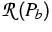of all probabilities on the path space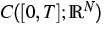which have the same bridges as the following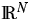-valued reference Brownian diffusion denoted by Pb and law of the solution of the stochastic differential system: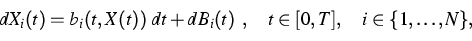where the drift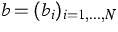is a regular function on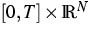.
The classis called the reciprocal class of the reference diffusion Pb. The random processes inare Markovian fields with respect to time and therefore they are Gibbsian fields associated with a time parameter belonging to the compact interval [0,T]. We prove in  that, under the assumption of finite entropy, the set of probability measures in the reciprocal class of Pb is characterized as the set of probability measures Q under which the following system of functional equations holds: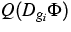=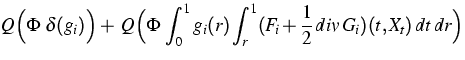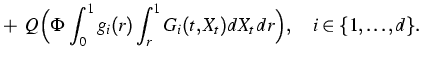(1)
Here,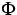is any regular test functional on, gi any step function on [0,T] with vanishing integral, Dgi denotes the Malliavin derivation operator in the direction gi;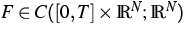and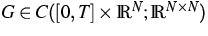are functions called reciprocal characteristics associated to Pb, and defined as follows: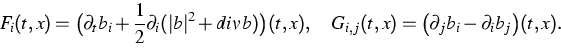Equation (1) is a perturbation of the duality equation satisfied by Brownian bridges, duality between the Malliavin derivation operator and the stochastic integral. The perturbation terms (second and third terms in the RHS of equation (1) ) are to be compared with the Malliavin derivatives of the Hamiltonian function associated to Gibbs measures. The main difference from the one-dimensional situation studied before in  comes from the new last term in (1), the stochastic integral of the reciprocal characteristic G w.r.t. the coordinate process. This term vanishes if and only if the drift b of the reference Brownian diffusion is a gradient. In  this term was identically zero since each regular function is a gradient in dimension N=1.
One finds in  several applications of this characterization of reciprocal processes. Among others, the authors prove a generalization of the famous Kolmogorov theorem about reversible diffusion: the existence of a reversible law in the reciprocal class of a Brownian diffusion with drift b can only occur if b is a gradient. In collaboration with L. Zambotti, they also study an application of the duality equation (1) in the singular case of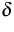-Bessel processes. There the difficulty consists in generalizing the reciprocal characteristics F and G associated to the degenerate drift function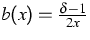.
The infinite-dimensional situation, when the index i gets continuous (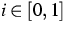) and the reference process is solution of a stochastic partial differential equation, is the next step in the study of such topics. It will be the subject of a forthcoming paper.

With D. Dereudre  we study Gibbsian properties on the path level of continuous systems of infinite-dimensional interactive Brownian diffusion. More precisely we consider the law of the solution of the following stochastic differential system with values in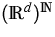: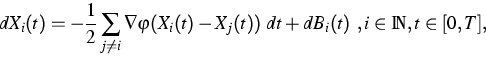(2)
where (Bi)i is a family of d-dimensional independent Brownian motions, and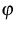is a bounded regular symmetrical potential with finite range. Such systems were introduced and solved first by R. Lang in . Since the coordinates of the process are indistinguishable, a solution of (2) can be represented by the point measure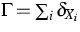on the path space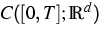, and the law of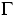, denoted by Q, is then a point process--or a field--on. We prove the equivalence between the following assertions: to be a Gibbsian field associated to an explicit Hamiltonian functional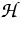on, and to be solution of the infinite-dimensional system (2) with a fixed Gibbsian initial condition. To this aim, a new characterization (cf. ) of canonical Gibbs fields on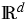andas solutions of duality formulae is used. Several applications are deduced from the Gibbsian structure on the path level of Q, like, in particular, the behavior of Q under time reversal (reversible and non-reversible cases are treated).

In collaboration with P. Dai Pra and H. Zessin , we give a Gibbsian characterization for the stationary law of the interacting diffusion process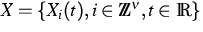defined on the latticeand solution of the following type of stochastic differential system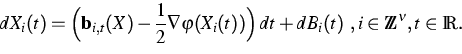(3)
The originality here is the fact that the interaction drift function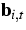is a very general measurable bounded local functional on the path space, a priori non-Markovian. The authors could interpret the law Q of X as a space-time Gibbs distribution on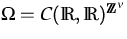. The reference specification is given as the infinite product of the bridges of the Wiener measure drifted by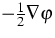, and the interaction potential is a certainon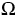given in terms of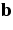.

In , based on the Gibbsian characterization shown in , a weak existence result for the solution of (3) is proved. The authors use a space-time cluster-expansion method, which is powerful when the coupling parameteris sufficiently small. As conclusion, the Gibbsian approach to study infinite-dimensional processes seems to be very effective in situations where the stochastic calculus can not give an answer, like for example in the question of existence of solution for the non-Markovian equation (3).

Consider now the following model of Statistical Physics in random medium: Langevin dynamics for a ferromagnetic system submitted to a disordered external Bernoulli magnetic field: for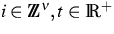,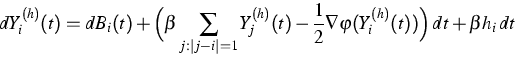(4)
where the (hi)i are Bernoulli i.i.d. variables. It is proved in  that such a system obeys a Strong Law of Large Numbers, in the sense that its empirical process converges towards a Dirac mass concentrated at some asymptotic dynamics Q, characterized as the law of the non-Markovian process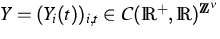solution of the following stochastic differential system: for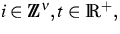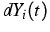=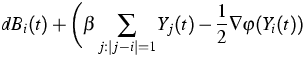(5) +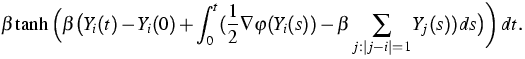In  the authors use the cluster-expansion method to show that the space-time correlation functions associated to Y decay exponentially fast in the high-temperature regime. In particular, they prove that for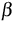small enough, the infinite-dimensional process Y(t) is ergodic and the velocity of the convergence is exponential. The specific difficulty of this model (compared to the system (3)) comes from the fact that the non-Markovian interaction (last term in the RHS of (5)) is no more local in time: it depends on the values of Y on the full time interval [0,t].

The process studied in  arises as diffusion limit of branching particle systems. It is called catalytic super-Brownian motion, in the sense that the branching procedure in the approximation depends strongly on a singular medium called catalyst. It is modeled as solution of the following stochastic partial differential equation: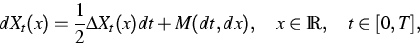(6)
where M is a martingale measure with quadratic variation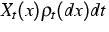, with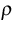a given family of singular measures like Cantor measures. H. Zähle studies in  the regularity of the function-valued solution of (6). The case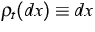is well known and corresponds to the situation when the catalyst is not singular, like for the Dawson-Watanabe superprocess. The type of generalization presented in  is new. The modulus of continuity in time and space of the function-valued solution of (6) is expressed in terms of the Hausdorff dimension of the support of the measure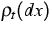.

References:

1. G. BEN AROUS, M. SORTAIS, Large deviations in the Langevin dynamics of the Random Field Ising Model, Preprint, Technische Universität Berlin, 2002, to appear in: Stochastic Process. Appl.
2. D. DEREUDRE, Une caractérisation de champs de Gibbs canoniques suret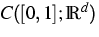, C.R. Acad. Sci. Paris Sér. I Math., 335 (2002), pp. 177-182.
3.,Diffusions infini-dimensionnelles et champs de Gibbs sur l'espace des trajectoires continues, PhD thesis, École Polytechnique, Paris, France, 2002.
4. P. DAI PRA, S. R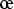lly, An existence result for infinite-dimensional Brownian diffusions with non-regular and non Markovian drift, to appear in: Markov Proc. Rel. Fields.
5. P. DAI PRA, S. Rlly, H. ZESSIN, A Gibbs variational principle in space-time for infinite dimensional diffusions, Probab. Theory Related Fields, 122 (2002), pp. 289-315.
6. R. LANG, Unendlich-dimensionale Wienerprozesse mit Wechselwirkung, Z. Wahrsch. verw. Gebiete, 39 (1977), pp. 55-72.
7. S. Rlly, M. SORTAIS, Space-time asymptotics of an infinite-dimensional diffusion having a long-range memory, WIAS Preprint no. 801, 2002.
8. S. Rlly, M. THIEULLEN, A characterization of reciprocal processes via an integration by parts formula on the path space, Probab. Theory Related Fields, 123 (2002), pp. 97-120.
9.,Duality formula for the bridges of a Brownian diffusion. Application to gradients drifts, WIAS Preprint no. 796, 2002.
10. H. ZÄHLE, Space-time regularity of catalytic super-Brownian motion, WIAS Preprint no. 783, 2002.

 [Next]: Stochastic models and numerical algorithms for
 [Up]: Project descriptions
 [Previous]: Probabilistic Cellular Automata
[Contents]   [Index]

LaTeX typesetting by I. Bremer
5/16/2003